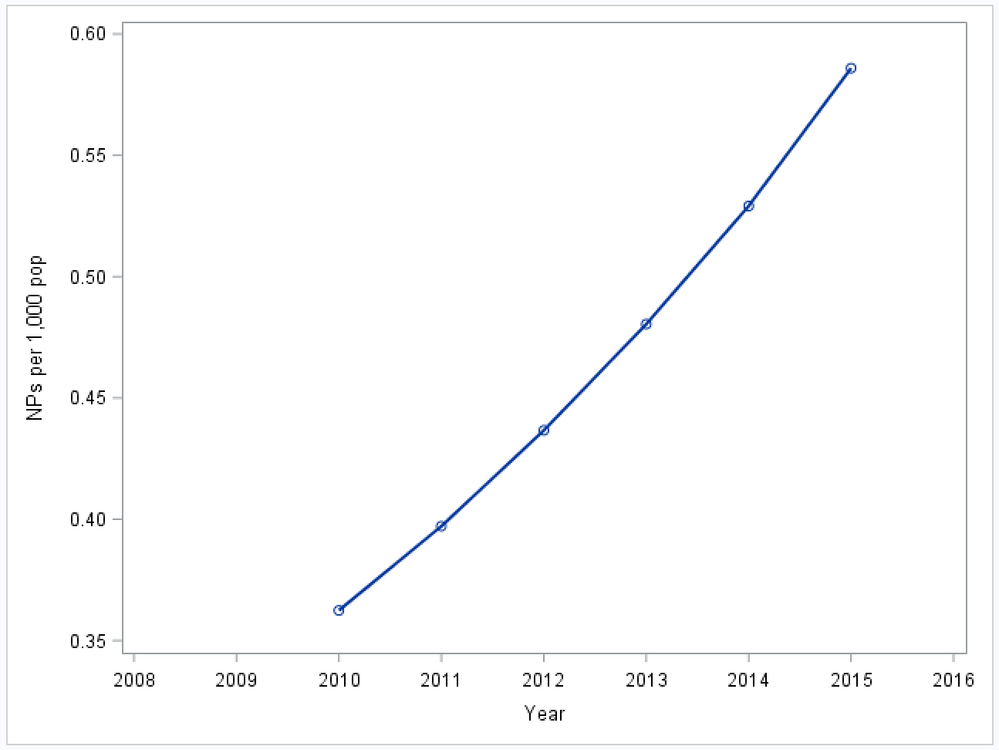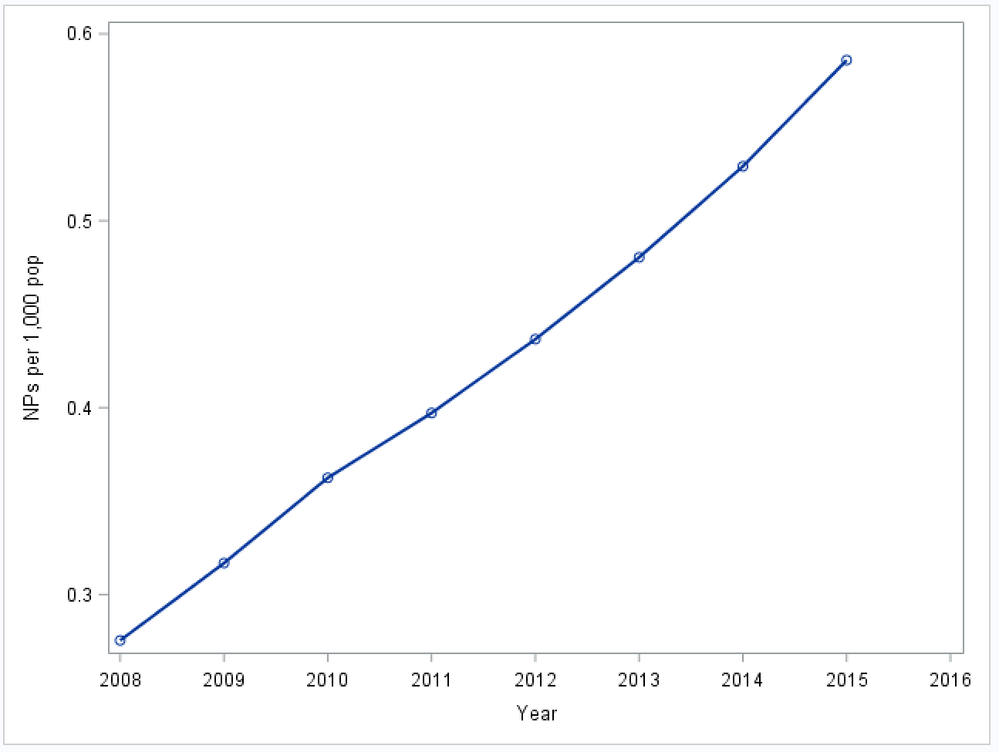## How to extrapolate or forecast values based on a fitted curve of existing values

I'd like to extrapolate missing values based on a fitted curve of existing data points, using version 9.4.  Here's my data:

Year    NPs_per_pop
2008    .
2009    .
2010    0.3624
2011    0.3971
2012    0.4366
2013    0.4804
2014    0.5291
2015    0.5859

Graphing the data looks like this:I'd like to estimate 2008 and 2009 based on a fitted curve of the existing 2010-2015 values.  Since I couldn't figure out how to "forecast" into the past, I first reversed the order of the data set, so that the first observation is from 2015 and the last is from 2008:

Order    NPs_per_pop
1           0.5859
2           0.5291
3           0.4804
4           0.4366
5           0.3971
6           0.3624
7           .
8           .

The closest I've been able to come is estimating based on the straight line of best fit, using proc esm:

``````proc esm data=original out=estimated lead=2;
forecast NPs_per_pop / model=linear;
run;``````

which produces this (when the order is reversed again, so it goes from 2008-2015):The linear model is an ok fit, but not great..... one reason is that the values should always be positive, fitting more of an exponential model.  I tried the different options of proc esm (e.g., transform=logistic), but nothing seemed to populate 2008 and 2009 with a fitted curve.  Any help on how to do that would be appreciated!

1 ACCEPTED SOLUTION

Accepted Solutions

## Re: How to extrapolate or forecast values based on a fitted curve of existing values

The SSM procedure handles a variety of models and it's syntax and output might take some getting used to.  You can see Example 3 ("Backcasting, Forecasting, and Interpolation") in the SSM doc for an additional example.  If you just want the back-casted values in your case, you can use the following modification of the code (print=smooth option in the MODEL statement):

proc ssm data=test;
id year interval=year;
trend curve(ps(2));
irregular wn;
model y = curve wn / print=smooth;
output out=for press;
run;

7 REPLIES 7Ksharp
Super User

## Re: How to extrapolate or forecast values based on a fitted curve of existing values

You could try PROC LOESS

or

proc reg data=have;

model y= x x^2 ;

quit;

@Rick_SAS wrote a blog about it before .

## Re: How to extrapolate or forecast values based on a fitted curve of existing values

Thanks for you response.

Proc reg gave me an error under the exponent operator (**), saying "ERROR 22-322: Syntax error, expecting one of the following: a name, ;, -, /,:, _ALL_, _CHARACTER_, _CHAR_, _NUMERIC_, {."

``````proc reg data=reg;
model NPs_per_pop = year year**2;
quit;``````

I tried the syntax of proc loess in one of the examples given, but it wouldn't produce a smoothed plot of my variables:

``````ods graphics on;
proc loess data=reg;
ods output OutputStatistics = Fit
FitSummary=Summary;
model NPs_per_pop = year / degree=2 select=AICC(steps) smooth = 0.6 1.0
direct alpha=.01;
run;
ods graphics off;``````

Either way, though, neither procedure seems to produce an extrapolation of missing data points.  Could you give a little more detail on how you were thinking those procedures would fill in missing values based on a fitted curve?

## Re: How to extrapolate or forecast values based on a fitted curve of existing values

Like PROC ESM, PROC SSM is also part of SAS/ETS.  You could use it for model based back-propagation, interpolation and forecasting.  It is a bit more involved than ESM.  Anyway, here is one possibility:

data test;
input year y@@;
year = mdy(1,1, year);
format year date.;
datalines;
2008    .
2009    .
2010    0.3624
2011    0.3971
2012    0.4366
2013    0.4804
2014    0.5291
2015    0.5859
;
proc ssm data=test;
id year interval=year;
trend curve(ps(2));
irregular wn;
model y = curve wn;
output out=for press;
run;
proc sgplot data=for;
scatter x=year y=y;
series x=year y=smoothed_curve;
reg x=year y=y;
run;

See the attached fit.

## Re: How to extrapolate or forecast values based on a fitted curve of existing values

Thanks for your response.  It looks like my computer doesn't have sufficient memory for proc ssm, though.  I got the following error message:

``````8485  proc ssm data=reg;
8486     id year interval=year;
8487     trend curve(ps(2));
8488     irregular wn;
8489     model NPs_per_pop = curve wn;
8490     output out=for press;
8491  run;

ERROR: Insufficient memory for data reading.``````

I'll try to find a computer with more memory to run the code you suggested. Thanks again.

## Re: How to extrapolate or forecast values based on a fitted curve of existing values

That is very strange.  SSM should not have memory issues for such a small sized problem even on a basic computer.  Anyway, keep me posted.

## Re: How to extrapolate or forecast values based on a fitted curve of existing values

The SSM procedure handles a variety of models and it's syntax and output might take some getting used to.  You can see Example 3 ("Backcasting, Forecasting, and Interpolation") in the SSM doc for an additional example.  If you just want the back-casted values in your case, you can use the following modification of the code (print=smooth option in the MODEL statement):

proc ssm data=test;
id year interval=year;
trend curve(ps(2));
irregular wn;
model y = curve wn / print=smooth;
output out=for press;
run;Ksharp
Super User

## Re: How to extrapolate or forecast values based on a fitted curve of existing values

``````data test;
input year y@@;
datalines;
2008    .
2009    .
2010    0.3624
2011    0.3971
2012    0.4366
2013    0.4804
2014    0.5291
2015    0.5859
;

ods output sgplot=temp;
proc sgplot data=test;
reg x=year y=y/cli clm degree=2;
run;

proc print noobs;run;
``````
Discussion stats
• 7 replies
• 2989 views
• 0 likes
• 3 in conversation